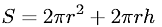Equations > Geometry > Area > Surface area of a cylinder

### Surface area of a cylinderLatex Code:

MathML Code:

 $S=2\pi {r}^{2}+2\pi \mathrm{rh}$

MathType 5.0: